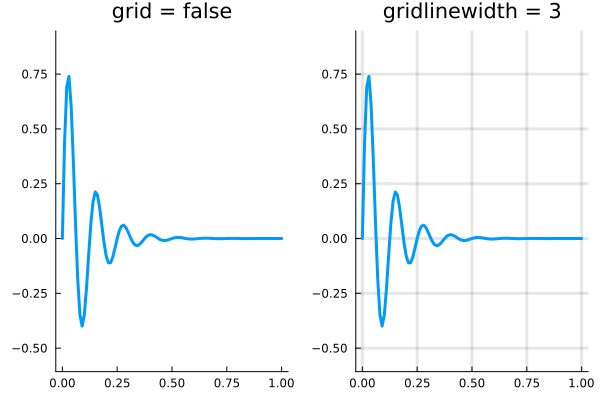## Grid Lines

There are a few attributes related to the grid lines.

Series attributesType
gridboolean
gridlinewidthNumber
gridalphaNumber in [0,1]
gridstyle:solid, :dash, :dot, :dashdot, :dashdotdot

In particular, setting

grid=false

will turn the grid lines off entirely, while something like In particular, setting

gridlinewidth = 3

will make the grid lines thicker than the default value.using Plots
x = 0:0.01:1;
y = @. sin(8*2π*x)/exp(10*x);

p1 = plot(
x,y,
lw=3,
label=false,
aspect_ratio = :equal,
title="grid = false",
grid=false
)

p2 = plot(
x,y,
lw=3,
label=false,
aspect_ratio = :equal,
title="gridlinewidth = 3",
gridlinewidth=3
)

plot(p1,p2,layout=(1,2))